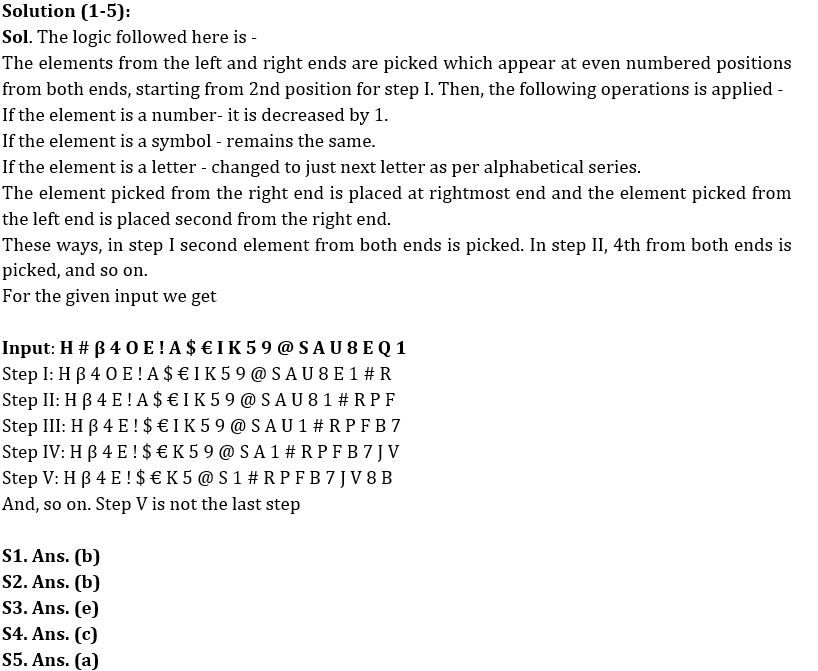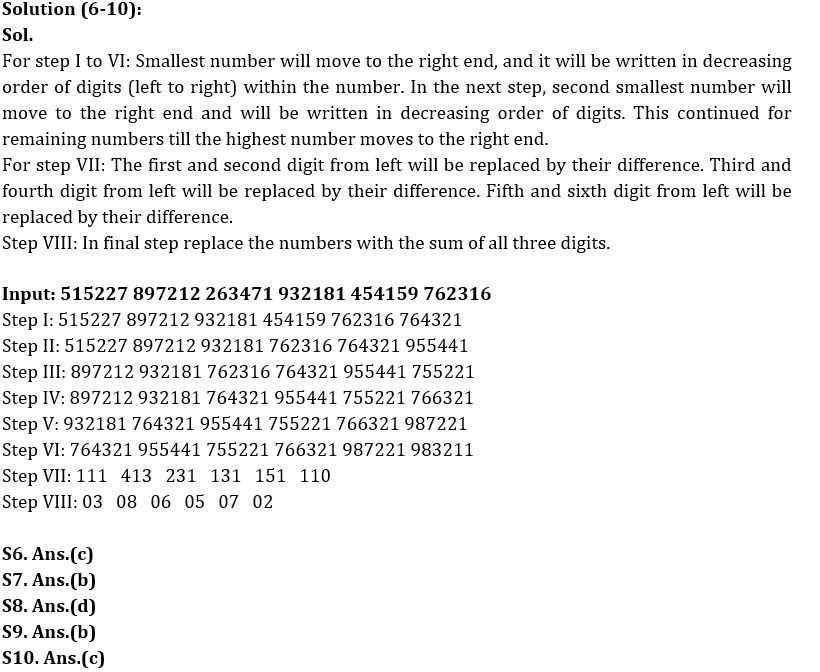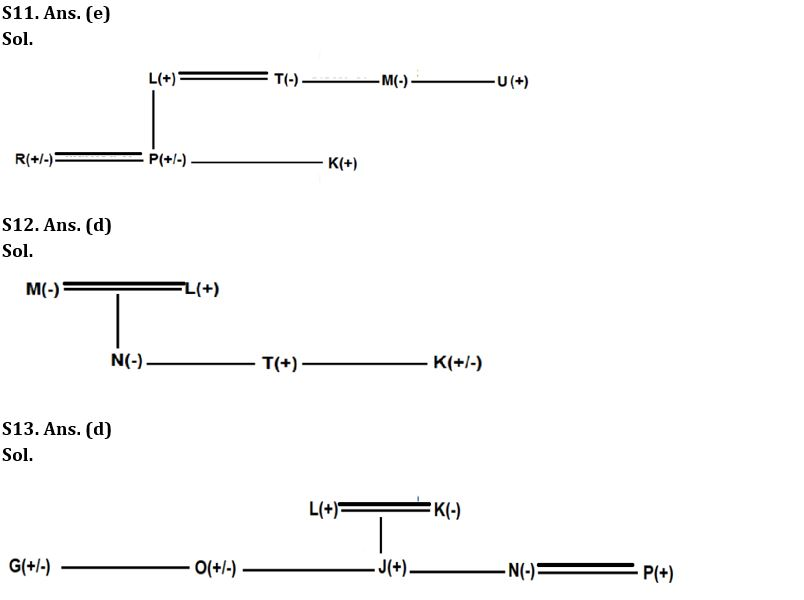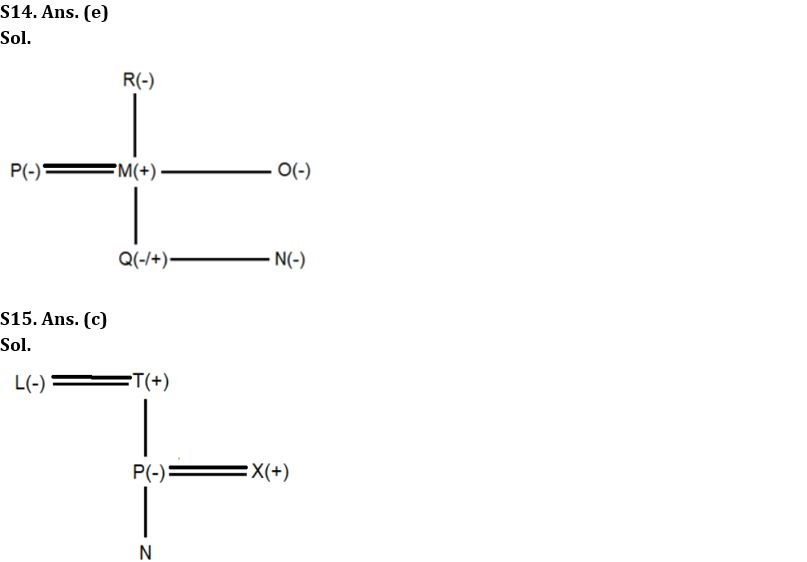Latest Banking jobs   »

# Reasoning Ability Quiz For SBI Clerk Mains 2022- 23rd December

Directions (1-5): When a letter/number/symbol arrangement machine is given an input line of letters/numbers/symbol, it arranges them following a certain rule. Following is an illustration of Input and rearrangement. Read the following information and answer the questions that follow

Input: A O \$ @ 5 H I % Q 2 4 E A K 9 > P U E # S ^ *
Step I: A \$ @ 5 H I % Q 2 4 E A K 9 > P U E # S * P ^
Step II: A \$ @ H I % Q 2 4 E A K 9 > P U E # * P ^ 4 T
Step III: A \$ @ H I Q 2 4 E A K 9 > P U E * P ^ 4 T % #
Step IV: A \$ @ H I Q 2 E A K 9 > P U * P ^ 4 T % # 3 F

Since there is no end to the number of steps of the above input one can make as many steps as intended. As per the rules followed in the above input, work out the steps for the following input:

Input: H # β 4 O E ! A \$ € I K 5 9 @ S A U 8 E Q 1

The number of steps to be worked out is more than four.

Q1. How many vowels appear in step V of the given input?
(a) None
(b) One
(c) Two
(d) Three
(e) More than three

Q2. In which of the following given steps, the last element is not a number?
(i) Step II
(ii) Step III
(iii) Step V
(iv) Step IV
(a) Only (ii)
(b) (i), (iii) and (iv)
(c) Only (iii)
(d) Both (ii) and (iv)
(e) All the given

Q3. Which element is third to the right of symbol ‘@’ in step IV?
(a) A
(b) R
(c) K
(d) #
(e) None of these

Q4. How many elements are between ‘β’ and ‘@’ in step II?
(a) Nine
(b) Eight
(c) Ten
(d) Seven
(e) None of these

Q5. Which of the following element is 4th to the left of 12th element from the right end in step IV?
(a) €
(b) !
(c) β
(d) E
(e) None of these

Directions (6-10): A number arrangement machine when given an input line of numbers rearranges them following a particular rule in each step. The following is an illustration of an input rearrangement.

Input: 356836 599832 137223 612573 213743 436587
Step I: 356836 599832 612573 213743 436587 733221
Step II: 356836 599832 612573 436587 733221 743321
Step III: 599832 612573 436587 733221 743321 866533
Step IV: 599832 612573 733221 743321 866533 876543
Step V: 612573 733221 743321 866533 876543 998532
Step VI: 733221 743321 866533 876543 998532 765321
Step VII: 411 301 210 111 031 121
Step VIII: 06 04 03 03 04 04

And the step VIII is the last step of the rearrangement. As per the rules followed in the above step, find out each of the following questions the appropriate step for the given input.

Input: 515227 897212 263471 932181 454159 762316

Q6. How many steps are required to get the final step of the given input?
(a) Six
(b) Seven
(c) Eight
(d) Nine
(e) None of the above

Q7. How many numbers are smaller than ‘6’ in the final step of the given input?
(a) Two
(b) Three
(c) Four
(d) Five
(e) More than five

Q8. Which of the following is the second last step of the given input?
(a) 112 413 231 131 151 110
(b) 111 413 234 131 151 110
(c) 111 413 231 131 152 110
(d) 111 413 231 131 151 110
(e) None of the above

Q9. What is the sum of all the digits of the fourth number from the left end in step IV of the given input?
(a) 25
(b) 28
(c) 32
(d) 33
(e) None of these

Q10. What is the sum of the numbers which are 4th from the right end and 2nd from the left end in step VIII?
(a) 12
(b) 16
(c) 14
(d) 10
(e) None of these

Directions (11-13): Answer the questions based on the information given below.
If A @ B means A is married to B,
A # B means B is sister of A,
A \$ B means B is father of A,
A & B means A is mother of B,
A % B means B is brother of A

Q11. In expression R @ P % K \$ L @ T # M % U, how is P related to U’s sister?
(a) Nephew
(b) Niece
(c) Son
(d) Daughter
(e) Cannot be determined

Q12. Which of the following expression will make T is brother of N definitely correct?
(a) K\$T@L&N#M
(b) T&K#N@L\$M
(c) Both (a) and (b)
(d) M&K#N%T\$L
(e) None of the above

Q13. If expression G%J\$L@K&O#N@P is true, then which of the following expressions is definitely correct?
(a) J#O
(b) G\$K
(c) P#N
(d) N\$L
(e) All of the above

Directions (14-15): Answer the questions based on the information given below.
In certain code,
A%B means A is father of B.
A* B means A is wife of B.
A@B means B is husband of A.
A#B means A is mother of B.
A&B means B is sister of A.
A+B means B is brother of A

Q14. If ‘R#M&P’ and O*M%Q&N is true then how is Q related to P?
(a) Nephew
(b) Father
(c) Mother
(d) Niece
(e) Cannot be determined

Q15. If L is grandmother of N then which of the following is correct?
(a) D*B%N%L
(b) K*M%N&L
(c) L@T%P*X%N
(d) C#S%L%N
(e) None of the above

Solutions#### Congratulations!Union Budget 2023-24: Free PDF